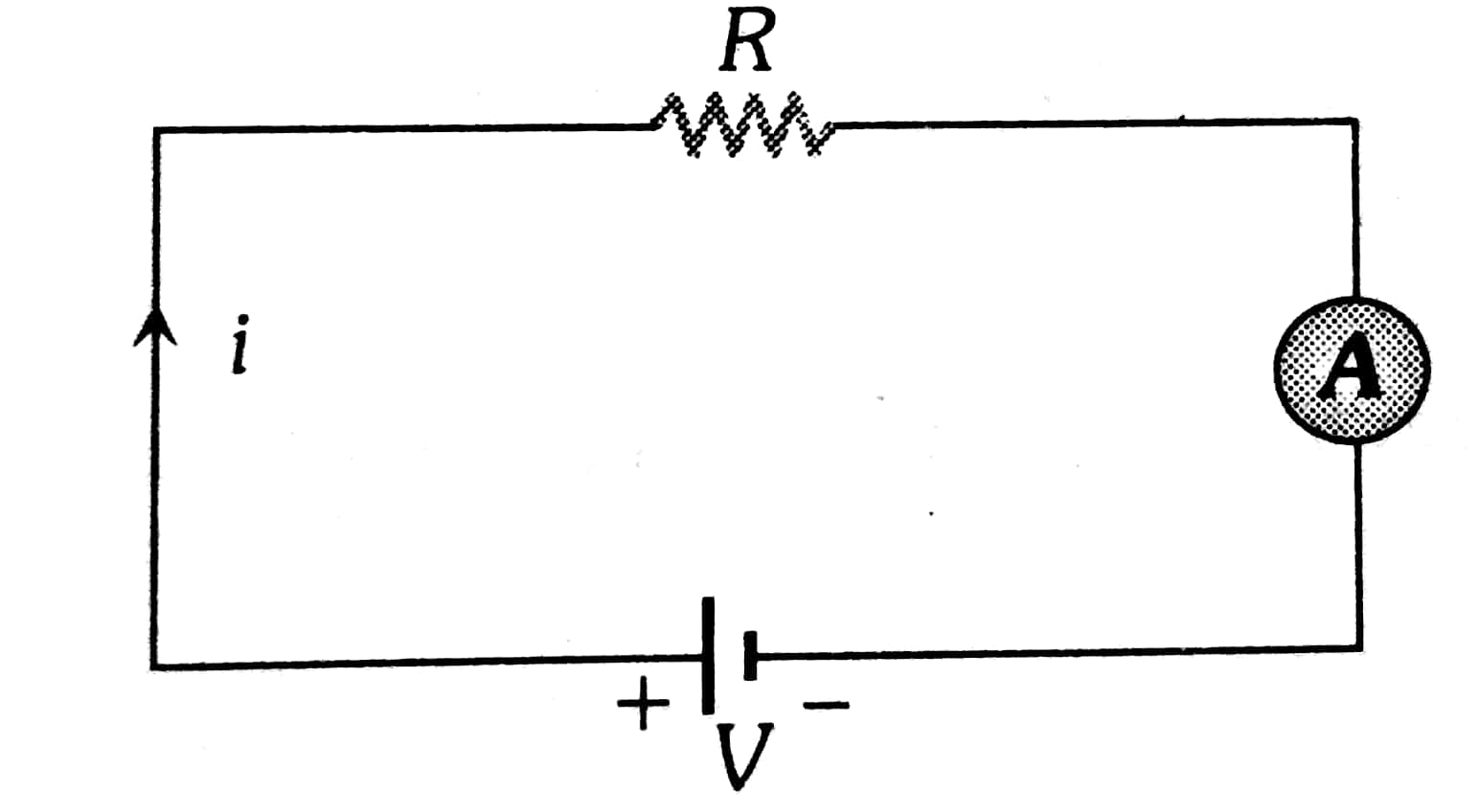Q

# Solve this problem A circuit contains an ammeter, a battery of 30 V and a resistance 40.8 ohm all connected in series. If the ammeter has a coil of resistance 480 ohm and a shunt of 20 ohm, the reading in the ammeter will be:

A circuit contains an ammeter, a battery of 30 V and a resistance 40.8 ohm all connected in series. If the ammeter has a coil of resistance 480 ohm and a shunt of 20 ohm, the reading in the ammeter will be:

• Option 1)

0.25 A

• Option 2)

2 A

• Option 3)

1 A

• Option 4)

0.5 A

726 Views

As we discussed in

Ammeter -

It is a device used to measure current and always connected in series

- whereinAs ammeter is in series with 40.8 Ω

Total resistance of the circuit

R=40.8ΩΩ+19.2Ω

From omni's law

Option 1)

0.25 A

This is incorrect option

Option 2)

2 A

This is incorrect option

Option 3)

1 A

This is incorrect option

Option 4)

0.5 A

This is correct option

Exams
Articles
Questions